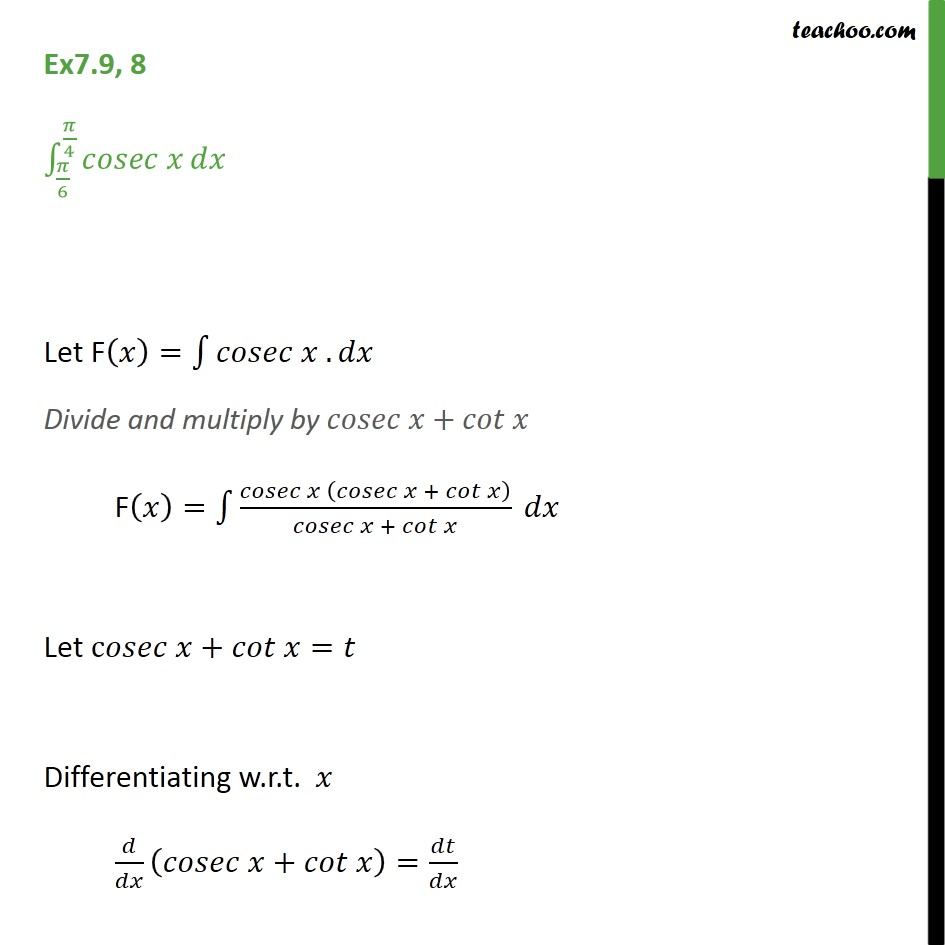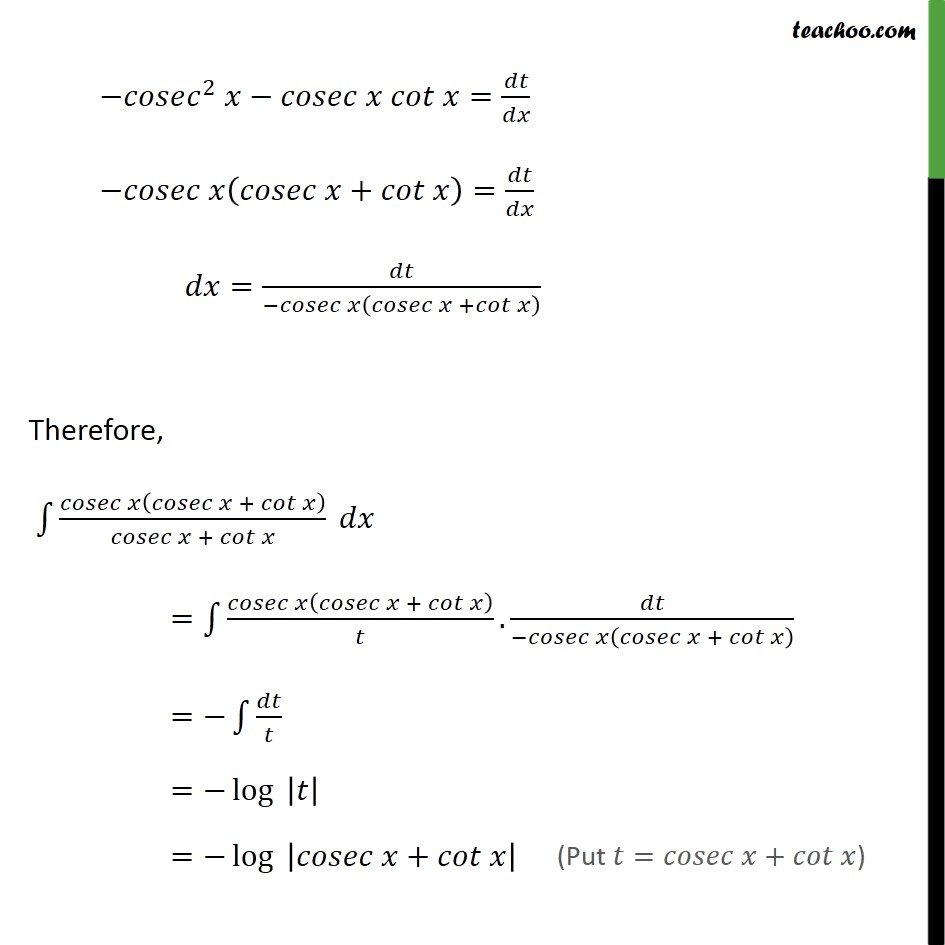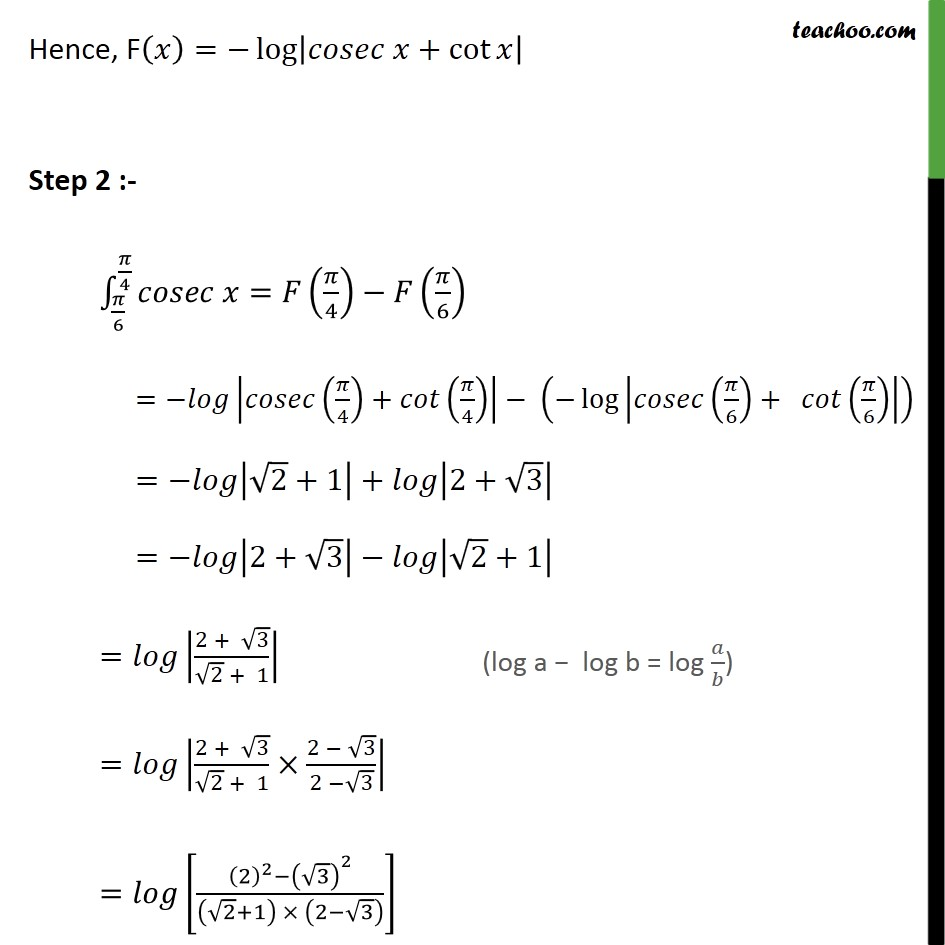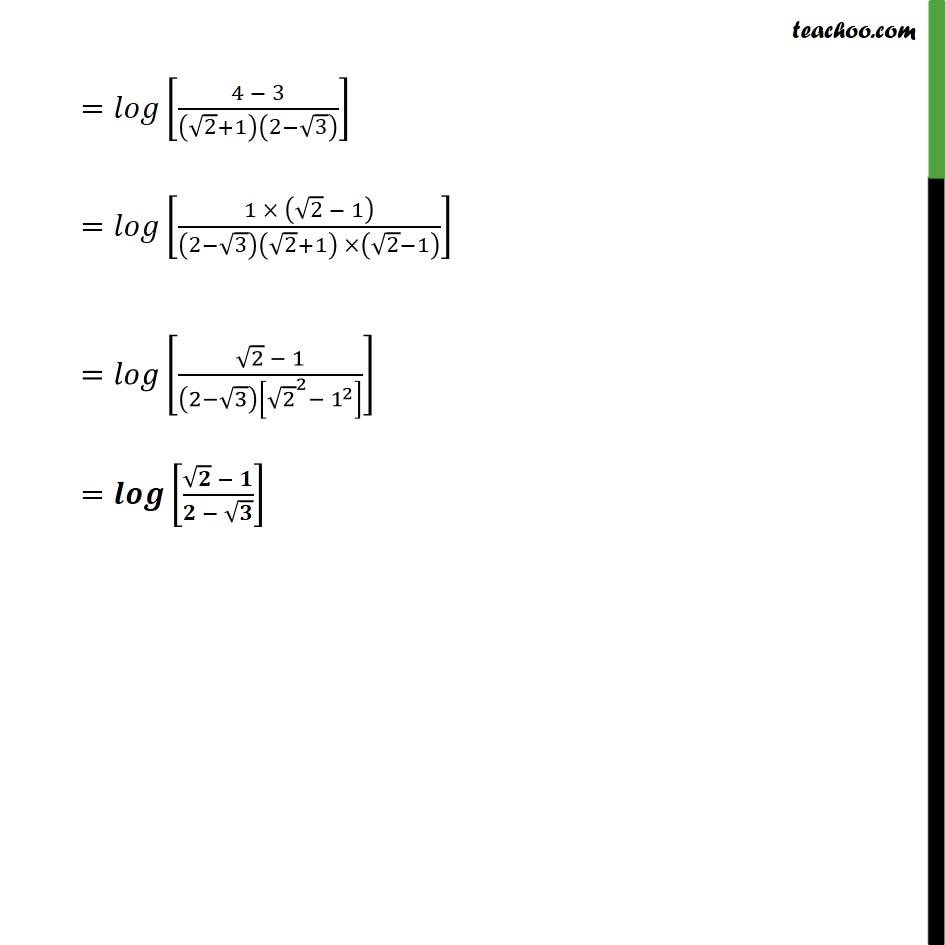1. Chapter 7 Class 12 Integrals
2. Serial order wise
3. Ex 7.9

Transcript

Ex7.9, 8 6 4 Let F = . Divide and multiply by + F = + + Let c + = Differentiating w.r.t. + = 2 = + = = + Therefore, + + = + . + = = log = log + Hence, F = log + cot Step 2 :- 6 4 = 4 6 = 4 + 4 log 6 + 6 = 2 +1 + 2+ 3 = 2+ 3 2 +1 = 2 + 3 2 + 1 = 2 + 3 2 + 1 2 3 2 3 = 2 2 3 2 2 +1 2 3 = 4 3 2 +1 2 3 = 1 2 1 2 3 2 +1 2 1 = 2 1 2 3 2 2 1 2 =

Ex 7.9

Chapter 7 Class 12 Integrals
Serial order wise